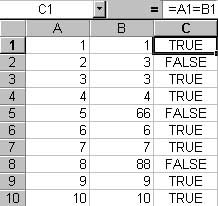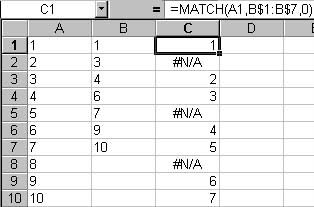## Friday, November 25, 2005

### Excel Row Matching

I see a lot of requests for information regarding how to match rows in Excel. Fortunately, there are several approaches for row matching in Excel. I tend to look at matching in two general categories, matching rows that are side by side, and matching rows to any value in a target column.

The first one is easy. Sort both columns, insert an indicating column, then use a simple formula like so:

“=A1 = C1”

The result is a Boolean value indicating a match has occurred.Figure 1: Match side by side rows using a formula

The second category can be done in several ways. VLOOKUP, which has the tendency to be a big PITA, or its less powerful cousin, MATCH. Unlike VLOOKUP, which allows you to return a value based on a column match (example: VLOOKUP will return the value in column D where column C matches the value in column A), MATCH will return the row number in a target range where the source value is found. If there is no match, the function returns “#N/A”.Figure 2: Match rows using the worksheet MATCH function.

There are times where you will need to do a match programmatically in VBA rather than using worksheet functions. It is possible to use Excels objects to get values from worksheet functions, or you could use an approach like the below macro, which can easily be modified to return the values in row, the row number, or a simple indicator letting the user know a match has occurred.

Sub Match_Rows()
'CONSTS-----------------------------------------------------------
'Define the row to start on to take into account header rows
Const START_ROW As Integer = 2

'VARIABLES--------------------------------------------------------
'x is a generic counter
Dim x As Integer
'Source, destination, and column to put X indicatiors columns
Dim srcColumn As String
Dim matchColumn As String
Dim dstColumn As String
'Variable to store the number of rows to process
Dim LastRow As Integer
'Used as a temporary object to indicate a match was found
Dim found_cell As Range
'Value to match
Dim matchValue As String

'CODE-------------------------------------------------------------
'Get the number of rows in this worksheet by using Excels internal countA function
'I use this instead of looking for blank cells because this has shown itself to be more accurate
If WorksheetFunction.CountA(Cells) > 0 Then
LastRow = Cells.Find(What:="*", After:=[A1], _
SearchOrder:=xlByRows, _
SearchDirection:=xlPrevious).Row
End If

'If there are no rows, exit out of the function
If LastRow = 0 Then
MsgBox "There are no rows in this worksheet", vbOKOnly
Exit Sub
End If

'Prompt the user for the source column containint employee IDs and the destination
'column indicating that the employee is active
srcColumn = InputBox("Enter the Source Column: ")
matchColumn = InputBox("Enter the Match Target Column: ")
dstColumn = InputBox("Enter the Results column: ")

'Set our generic counter to the start row defined in the constants section
x = START_ROW

'Loop from our starting row to the last row, on error use the general exception handler
On Error GoTo Error_Occured
For x = START_ROW To LastRow
'Get the value to match
matchValue = Cells(Val(x), Trim(UCase(srcColumn))).Value

'Select the match Column
Columns(UCase(matchColumn) & ":" & UCase(matchColumn)).Select

'Set the found_Cell object to an instance inside of the matching column
'that has a matching value
Set found_cell = Selection.Find(What:=matchValue, After:=ActiveCell, LookIn:= _
xlValues, LookAt:=xlWhole, SearchOrder:=xlByRows, SearchDirection:= _
xlNext, MatchCase:=False)

'If the object was found, set the target to "X", otehrwise set to blank
Range(Trim(UCase(dstColumn)) & Val(x)).Value = "X"
Else
Range(Trim(UCase(dstColumn)) & Val(x)).Value = ""
End If

'reset found_cell to nothing after each run
Set found_cell = Nothing
Next
Exit Sub
'The general exception handler
Error_Occured:
Set found_cell = Nothing
MsgBox "An error occured", vbOKOnly
End Sub